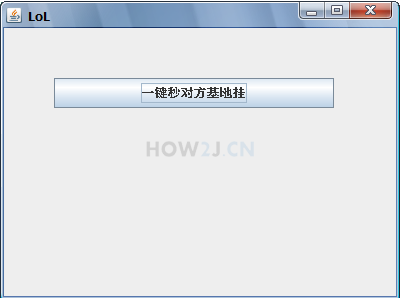GUI-Graphic User Interface 图形用户界面6分39秒

## 简单的例子

JFrame是GUI中的容器
JButton是最常见的组件- 按钮package gui; import javax.swing.JButton; import javax.swing.JFrame; public class TestGUI { public static void main(String[] args) { // 主窗体 JFrame f = new JFrame("LoL"); // 主窗体设置大小 f.setSize(400, 300); // 主窗体设置位置 f.setLocation(200, 200); // 主窗体中的组件设置为绝对定位 f.setLayout(null); // 按钮组件 JButton b = new JButton("一键秒对方基地挂"); // 同时设置组件的大小和位置 b.setBounds(50, 50, 280, 30); // 把按钮加入到主窗体中 f.add(b); // 关闭窗体的时候，退出程序 f.setDefaultCloseOperation(JFrame.EXIT_ON_CLOSE); // 让窗体变得可见 f.setVisible(true); } }

## 练习-在上次关闭位置启动窗口

姿势不对,事倍功半! 点击查看做练习的正确姿势

## 答案-在上次关闭位置启动窗口5分10秒 本视频采用html5方式播放，如无法正常播放，请将浏览器升级至最新版本，推荐火狐，chrome，360浏览器。 如果装有迅雷，播放视频呈现直接下载状态，请调整 迅雷系统设置-基本设置-启动-监视全部浏览器 (去掉这个选项)。 chrome 的 视频下载插件会影响播放，如 IDM 等，请关闭或者切换其他浏览器

package gui; import java.io.DataOutputStream; import java.io.File; import java.io.FileOutputStream; import javax.swing.JFrame; class SavingPostionThread extends Thread { private JFrame f; File file = new File("e:/project/j2se/location.txt"); SavingPostionThread(JFrame f) { this.f = f; } public void run() { while (true) { int x = f.getX(); int y = f.getY(); try (FileOutputStream fos = new FileOutputStream(file); DataOutputStream dos = new DataOutputStream(fos);) { dos.writeInt(x); dos.writeInt(y); } catch (Exception e) { e.printStackTrace(); } try { Thread.sleep(100); } catch (InterruptedException e) { // TODO Auto-generated catch block e.printStackTrace(); } } } }
package gui; import java.io.DataInputStream; import java.io.File; import java.io.FileInputStream; import java.io.FileNotFoundException; import java.io.IOException; import javax.swing.JButton; import javax.swing.JFrame; public class TestGUI { public static void main(String[] args) { // 主窗体 JFrame f = new JFrame("LoL"); // 主窗体设置大小 f.setSize(400, 300); // 主窗体设置位置 Point p =getPointFromLocationFile(); if(p!=null) f.setLocation(p.x,p.y); else f.setLocation(200, 200); // 主窗体中的组件设置为绝对定位 f.setLayout(null); // 按钮组件 JButton b = new JButton("一键秒对方基地挂"); // 同时设置组件的大小和位置 b.setBounds(50, 50, 280, 30); // 把按钮加入到主窗体中 f.add(b); // 关闭窗体的时候，退出程序 f.setDefaultCloseOperation(JFrame.EXIT_ON_CLOSE); // 让窗体变得可见 f.setVisible(true); new SavingPostionThread(f).start(); } static class Point { int x; int y; } public static Point getPointFromLocationFile() { File file = new File("e:/project/j2se/location.txt"); Point p = null; try (FileInputStream fis = new FileInputStream(file); DataInputStream dis = new DataInputStream(fis);) { int x = dis.readInt(); int y = dis.readInt(); p = new Point(); p.x = x; p.y = y; } catch (FileNotFoundException e) { //第一次运行，并没有生成位置文件，所以会出现FileNotFoundException } catch (IOException e1) { e1.printStackTrace(); } return p; } }

HOW2J公众号，关注后实时获知最新的教程和优惠活动，谢谢。2021-09-17 练习 - 在上次关闭位置启动窗口
 莫諾 比如这次使用这个窗口，导致窗口被移动到了右下角。 关闭这个窗口，下一次再启动的时候，就会自动出现在右下角。 思路提示： 启动一个线程，每个100毫秒读取当前的位置信息，保存在文件中，比如location.txt文件。 启动的时候，从这个文件中读取位置信息，如果是空的，就使用默认位置，如果不是空的，就把位置信息设置在窗口上。 读取位置信息的办法： f.getX() 读取横坐标信息，f.getY()读取纵坐标信息。 注：这个练习要求使用多线程来完成。```public class TestGUI { static int x = 5; static int y = 5; static int j = 300; static int k = 150; static JFrame f = new JFrame("LOL"); // 准备空文件location.txt static File f1 = new File("E:/project/j2se/src/location.txt"); static List ss =new ArrayList<>(); public static void main(String[] args) { TestGUI sx = new TestGUI(); //创建基于文件的输出流 自动创建目录以及文件 if (f1.exists() == false) { f1.getParentFile().mkdirs(); try { f1.createNewFile(); } catch (IOException e) { // TODO 自动生成的 catch 块 e.printStackTrace(); } } // 使用传统方式创建100个线程 int n = 100; Thread[] Threads = new Thread[n]; for (int i = 0; i < n; i++) { Thread t = new Thread(){ public void run(){ try { Thread.sleep(500); // 读取模板 String s1 = null; String x1 = String.valueOf(x); String y1 = String.valueOf(y); s1 = x1 + "," + y1; // 更新内容 ss.add(s1); // 把更新后的内容写入新文件 sx.write(ss,f1); if(sx.readTem(f1).length == 0) {; } else if(sx.readTem(f1).length > 0) { ;} sx.Frame(f); sx.StartFrame(f); // 位置已更新 Thread.sleep(500); sx.CloseFrame(f); } catch (InterruptedException e) { // TODO 自动生成的 catch 块 e.printStackTrace(); } } }; t.start(); Threads[i] = t; try { t.join(); } catch (InterruptedException e) { // TODO 自动生成的 catch 块 e.printStackTrace(); } } } public void Frame(JFrame f) { f.setSize(j, k); f.setLocation(x, y); f.setLayout(null); JButton b = new JButton("一键秒对方基地"); b.setBounds(50, 50, 200, 30); f.add(b); f.setDefaultCloseOperation(JFrame.EXIT_ON_CLOSE); } public void CloseFrame(JFrame f) { f.dispose(); } public void StartFrame(JFrame f) { x = f.getX(); y = f.getY(); f.setLocation(x, y); f.setVisible(true); x += j; y += k; } private void write(List ss,File f) { try ( FileWriter fw = new FileWriter(f); PrintWriter pw = new PrintWriter(fw); ) { for (int i = 0; i < ss.size(); i++) { pw.println(ss.get(i)); } pw.close(); fw.close(); } catch (IOException e) { e.printStackTrace(); } } public String[] readTem(File f){ int l = 0;int i = 0; try ( FileReader fr = new FileReader(f); BufferedReader br = new BufferedReader(fr); ) { while (true) { String line = br.readLine(); if (null == line) break; l++; } br.close(); fr.close(); } catch (IOException e) { e.printStackTrace(); } String[] s0= new String[l]; try ( FileReader fr = new FileReader(f); BufferedReader br = new BufferedReader(fr); ) { while (true) { String line = br.readLine(); if (null == line) break; s0[i] = line; i++; } br.close(); fr.close(); } catch (IOException e) { e.printStackTrace(); } return s0; } }```

2021-08-25 在上次关闭位置启动窗口
 logiczqr 练习 ```package gui; import java.io.BufferedReader; import java.io.File; import java.io.FileReader; import java.io.IOException; import java.io.PrintWriter; import javax.swing.JButton; import javax.swing.JFrame; public class TestGUI { public static final File FILE = new File("C:\\Users\\Administrator\\Desktop\\location.txt"); public static void main(String[] args) { // 主窗体 JFrame jf = new JFrame("LOL"); // 主窗体设置大小 jf.setSize(400, 300); // 主窗体设置位置 int[] xy = method2(); int x, y; if (xy != null) { x = xy; y = xy; jf.setLocation(x, y); } else { jf.setLocation(200, 200); } // 主窗体中的组件设置为绝对定位 jf.setLayout(null); // 按钮组件 JButton jb = new JButton("一键秒对方基地挂"); // 同时设置组件的大小和位置 jb.setBounds(50, 50, 280, 30); // 把按钮加入到主窗体中 jf.add(jb); // 关闭窗体的时候，退出程序 jf.setDefaultCloseOperation(JFrame.EXIT_ON_CLOSE); // 让窗体变得可见 jf.setVisible(true); method(jf); } /** * 把窗口位置信息写出到文件中 * @param jf */ public static void method(JFrame jf) { Thread thread = new Thread() { public void run() { while (true) { int x = jf.getX(); int y = jf.getY(); try (PrintWriter pw = new PrintWriter(FILE);) { pw.print(x + "@" + y); pw.flush(); Thread.sleep(100); } catch (IOException | InterruptedException e) { // TODO Auto-generated catch block e.printStackTrace(); } } } }; thread.start(); } /** * 读入文件中的位置信息 * @return */ public static int[] method2() { int[] is = null; try (FileReader fr = new FileReader(FILE); BufferedReader br = new BufferedReader(fr);) { String str = br.readLine(); String[] ss = str.split("@"); int[] is2 = new int; is2 = Integer.parseInt(ss); is2 = Integer.parseInt(ss); is = is2; } catch (IOException e) { // TODO: handle exception e.printStackTrace(); } return is; } } ```

2021-07-08 这是我的答案， 我测试通过了。
2021-04-29 打卡第一张
2021-04-18 为什么加到try（）里就不行 循环里就行